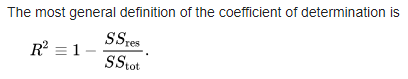# How can we infer R squared in ML course lesson 1In the definition of coefficient of determination, the denominator tells about “variance of train data”, but numerator tells about “error between expected and predicted”. How can we compare two different terms? How can more/less variance influence the residual error? If residual error is zero, then does that mean the model is bad, despite error is zero(which mean model fits perfectly).
Correct me if I interpret wrongly.

The numerator is proportional to the variance of the residual between the model and the data, while the denominator is proportional to the total variance of the data. Here, (1) residual means the difference between the predicated and actual y values, and (2) total variance is the mean of the squared differences in y values from their mean.

In the ideal case, the model fits perfectly, the residual SS_{res} is zero, and R^2 = 1, showing that the total variance in the data is completely accounted for by the model.

On the other hand if we are very lazy and just model the data as its mean, then SS_{res} is the same as SS_{tot} so the ratio is 1, and R^2=0

When the residual has nonzero variance, \frac{SS_{res}}{SS_{tot}} is the fraction of the total variance that is not explained by the model. This can be due to noise and/or having an incomplete model.
So R^2 = 1 - \frac{SS_{res}}{SS_{tot}} is the fraction of the total variance that is explained by the model, and it varies on a scale from 0 to 1, with 1 being the best.